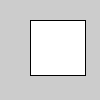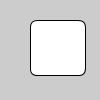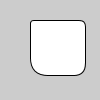Name

### rect()

Examples```rect(30, 20, 55, 55)
``````rect(30, 20, 55, 55, 7)
``````rect(30, 20, 55, 55, 3, 6, 12, 18)
```
Description Draws a rectangle to the screen. A rectangle is a four-sided shape with every angle at ninety degrees. By default, the first two parameters set the location of the upper-left corner, the third sets the width, and the fourth sets the height. The way these parameters are interpreted, however, may be changed with the rectMode() function.

To draw a rounded rectangle, add a fifth parameter, which is used as the radius value for all four corners.

To use a different radius value for each corner, include eight parameters. When using eight parameters, the latter four set the radius of the arc at each corner separately, starting with the top-left corner and moving clockwise around the rectangle.
Syntax
```rect(a, b, c, d)
rect(a, b, c, d, r)
rect(a, b, c, d, tl, tr, br, bl)
```
Parameters
a float: x-coordinate of the rectangle by default float: y-coordinate of the rectangle by default float: width of the rectangle by default float: height of the rectangle by default float: radii for all four corners float: radius for top-left corner float: radius for top-right corner float: radius for bottom-right corner float: radius for bottom-left corner
Related rectMode()